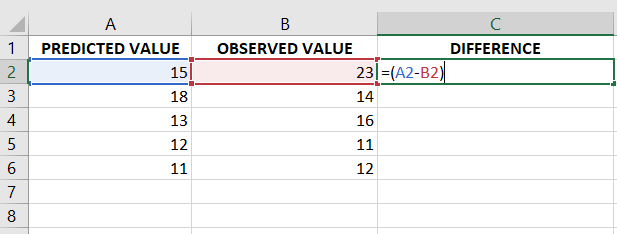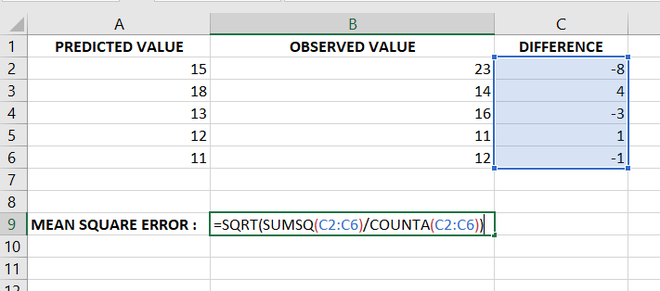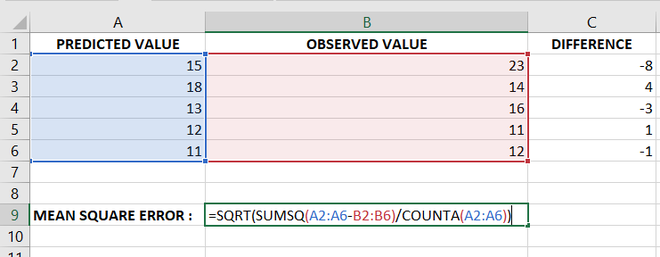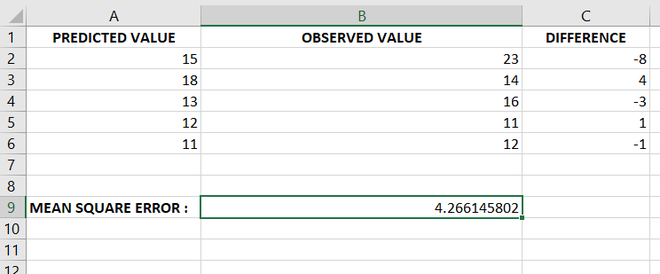# How to Calculate Root Mean Square Error in Excel?

• Last Updated : 23 Jun, 2021

In simple terms, Root mean square error means how much far apart are the observed values and predicted values on average. The formula for calculating the root-mean-square error is as follows :Where,

• n: number of samples
• f: Forecast
• o: observed values

## Calculating Root Mean Square Error in Excel :

Follow the below steps to calculate the root means square error in Excel:

• Fill up the predicted values, observed values, and differences between them in the Excel sheet.
• To calculate the difference, just type the formula in one cell and then just drag that cell to the rest of the cells. The difference between the cells will be calculated automatically. Then either follow STEP 3 or STEP 4 given below :Calculating the difference between the observed values and predicted values

• Now let us select one cell and apply the formula of root-mean-square error.Method 1: Formula to Calculate Root Mean Square Error (RMSE)

• We could have not used the difference column at all, we can directly calculate RMS error from Predicted and Observed values Columns as follows :Method 2: Formula to calculate Root Mean Square Error ( RMSE)

• At Last, we get the required Root Mean Square error value in the selected cell.Root mean square error is calculated in the selected cell after applying the formula

### Applications of Root Mean Square Error value in different domains :

Following are some applications of RMSE:

• It is used to predict how the atmosphere behaves and how it differs from the predicted behavior in the meteorology domain.
• It can be used to measure the average distance between two proteins that are superimposed on one other.
• It can be used to calculate the Peak Signal to Noise ratio in the field of image processing to determine the effectiveness of a method that reconstructs an image as compared to the original image.
My Personal Notes arrow_drop_up